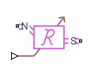# Variable Reluctance

Variable reluctance in electromagnetic systems

## Library

Magnetic Elements

•## Description

The Variable Reluctance block models a variable reluctance, that is, a component that resists flux flow. The ratio of the magnetomotive force (mmf) across the component to the resulting flux that flows through the component is defined as the reluctance, and is dependent on the value of the input physical signal.

The block is based on the following equations:

`$MMF=\Phi \cdot \Re$`
`$\Re =\frac{X}{{\mu }_{0}\cdot {\mu }_{r}\cdot A}$`

where

 MMF Magnetomotive force (mmf) across the component Φ Flux through the component $\Re$ Reluctance X Value presented at the physical signal port μ0 Permeability constant μr Relative permeability of the material A Cross-sectional area of the section being modeled

Connections N and S are magnetic conserving ports. The mmf across the reluctance is given by $MMF\left(N\right)-MMF\left(S\right)$, and the sign of the flux is positive when flowing through the device from N to S.

### Variables

To set the priority and initial target values for the block variables prior to simulation, use the Initial Targets section in the block dialog box or Property Inspector. For more information, see Set Priority and Initial Target for Block Variables.

Nominal values provide a way to specify the expected magnitude of a variable in a model. Using system scaling based on nominal values increases the simulation robustness. Nominal values can come from different sources, one of which is the Nominal Values section in the block dialog box or Property Inspector. For more information, see Modify Nominal Values for a Block Variable.

## Parameters

Minimum length or thickness X>=0

The minimum value of length of air gap or thickness of section. If the input signal falls below this level (for example, turns negative), this minimum value is used. The parameter value must be nonnegative. The default value is `0`.

Cross-sectional area

Area of the section being modeled. The default value is `0.01` m^2.

Relative permeability of material

Relative permeability of the section material. The default value is `1`.

## Ports

The block has the following ports:

`N`

Magnetic conserving port associated with the block North terminal.

`S`

Magnetic conserving port associated with the block South terminal.

The block also has one physical signal input port that provides the value of the length of air gap or thickness of section.

## Version History

Introduced in R2010a